RRB Group D Mock Test - 33

# RRB Group D Mock Test - 33

Test Description

## 100 Questions MCQ Test RRB (Group D) - Mock Tests & Previous Year Papers | RRB Group D Mock Test - 33

RRB Group D Mock Test - 33 for Railways 2023 is part of RRB (Group D) - Mock Tests & Previous Year Papers preparation. The RRB Group D Mock Test - 33 questions and answers have been prepared according to the Railways exam syllabus.The RRB Group D Mock Test - 33 MCQs are made for Railways 2023 Exam. Find important definitions, questions, notes, meanings, examples, exercises, MCQs and online tests for RRB Group D Mock Test - 33 below.
Solutions of RRB Group D Mock Test - 33 questions in English are available as part of our RRB (Group D) - Mock Tests & Previous Year Papers for Railways & RRB Group D Mock Test - 33 solutions in Hindi for RRB (Group D) - Mock Tests & Previous Year Papers course. Download more important topics, notes, lectures and mock test series for Railways Exam by signing up for free. Attempt RRB Group D Mock Test - 33 | 100 questions in 90 minutes | Mock test for Railways preparation | Free important questions MCQ to study RRB (Group D) - Mock Tests & Previous Year Papers for Railways Exam | Download free PDF with solutions
 1 Crore+ students have signed up on EduRev. Have you?
RRB Group D Mock Test - 33 - Question 1

### India's first Kisan Mandi is set up in?

RRB Group D Mock Test - 33 - Question 2

### India's first Kisan Mandi is set up in?

RRB Group D Mock Test - 33 - Question 3

### Which is the smallest moon in the solar system?

RRB Group D Mock Test - 33 - Question 4

A vote of no-confidence in India has to be started in …

RRB Group D Mock Test - 33 - Question 5

Which state has the highest number of dam in India?

RRB Group D Mock Test - 33 - Question 6

The minimum numbers of members in state legislative council?

RRB Group D Mock Test - 33 - Question 7

1st Lok Adalat was held in?Report Question

RRB Group D Mock Test - 33 - Question 8

Which of these diseases is related o memory loss?

RRB Group D Mock Test - 33 - Question 9

Which famous freedom fighter gave the slogan, 'Give me blood and I shall give you freedom'?

RRB Group D Mock Test - 33 - Question 10

Thermochemical decomposition of organic materials at high temperatures, in the absence of oxygen is called

RRB Group D Mock Test - 33 - Question 11

Who has been crowned the Miss World 2017?

RRB Group D Mock Test - 33 - Question 12

The Scheme launched by government which proposes to select 1,000 best B.Tech students each year from premier institutions and provide them with facilities to pursue Ph.D. in IITs and IISc with a handsome fellowship.

RRB Group D Mock Test - 33 - Question 13

Which of the following Indian Sportspersons did NOT win the Rajiv Gandhi Khel Ratna award?

Detailed Solution for RRB Group D Mock Test - 33 - Question 13

Sachin Tendulkar and MS Dhoni are the only two cricketers to win the highest sporting award in India, the Rajiv Gandhi Khel Ratna award.

RRB Group D Mock Test - 33 - Question 14

Who is the current Director General of Directorate of Civil Aviation?

RRB Group D Mock Test - 33 - Question 15

What is the major initiative taken up by the government to step up investments in research and related infrastructure in premier educational institutions, including health institutions?

RRB Group D Mock Test - 33 - Question 16

”Jab” is a term associated with which of the following?

RRB Group D Mock Test - 33 - Question 17

Who was the first recipient of the Rajiv Gandhi Khel Ratna?

RRB Group D Mock Test - 33 - Question 18

How many MB make a TB?

RRB Group D Mock Test - 33 - Question 19

What is the highest mountain peak in Europe?

RRB Group D Mock Test - 33 - Question 20

Who was the last Mughal Emperor of India?

RRB Group D Mock Test - 33 - Question 21

The wheel with 24 spokes at the centre of the Indian National Flag is taken from the edicts of which Indian king?

RRB Group D Mock Test - 33 - Question 22

The Southern Zone of the Indian Railways is Head Quartered at which of the following Places?

RRB Group D Mock Test - 33 - Question 23

The "Golden Chariot" a Luxury Tourist Train is jointly operated by Indian Railways and which of the following state Tourism Organisations?

RRB Group D Mock Test - 33 - Question 24

Which of the following terms is NOT used in the game of Cricket?

RRB Group D Mock Test - 33 - Question 25

Who is the current chairman of UPSC ?

RRB Group D Mock Test - 33 - Question 26

Which year was observed as the 'World Population Year' by United Nations?

RRB Group D Mock Test - 33 - Question 27

Which country is going to host winter Olympics 2022?

RRB Group D Mock Test - 33 - Question 28

Most rivers in North India are … and most rivers in South India are ….

RRB Group D Mock Test - 33 - Question 29

Which is the scheme launched by government aimed to boosting investment in food processing Sector?

RRB Group D Mock Test - 33 - Question 30

Which of the following persons is the current President of the Indian Olympic Association?

RRB Group D Mock Test - 33 - Question 31

Which of the following is the correct abbreviation of SEBI?

RRB Group D Mock Test - 33 - Question 32

Which famous Indian hockey player was called "the Wizard of Hockey"?

RRB Group D Mock Test - 33 - Question 33

Pick the true statements from the options given.

RRB Group D Mock Test - 33 - Question 34

The deficiency of red blood cells in blood leads to

RRB Group D Mock Test - 33 - Question 35

Sir CV Raman was awarded the Nobel Prize for his work on

RRB Group D Mock Test - 33 - Question 36

Who among the below was called the 'Father of Indian Renaissance’?

RRB Group D Mock Test - 33 - Question 37

The only Union Territory of India with three official regional languages is ….

RRB Group D Mock Test - 33 - Question 38

Which of these colours is having the highest wavelength?

RRB Group D Mock Test - 33 - Question 39

In which year was the prevention of terrorism act (POTA) enacted?

RRB Group D Mock Test - 33 - Question 40

Chathh Puja festival is celebrated in which state?

RRB Group D Mock Test - 33 - Question 41

What is the code name given to the operation by the Indian Peace Keeping Force (IPKF) sent to Sri Lanka by Rajiv Gandhi in 1987?

RRB Group D Mock Test - 33 - Question 42

Indian monsoons are often referred to as the Real …. of India.

RRB Group D Mock Test - 33 - Question 43

The name of the satellite launch station in Sriharikota, Andhra Pradesh is …

RRB Group D Mock Test - 33 - Question 44

In which year was the Rowlatt Act passed by British India?

RRB Group D Mock Test - 33 - Question 45

Which of the following factors doesn't impact the Process of Photosynthesis?

RRB Group D Mock Test - 33 - Question 46

What is the common name of sodium hypochlorite?

RRB Group D Mock Test - 33 - Question 47

Which is the only year in which Mahatma Gandhi presided over the annual session of the Indian National Congress?

RRB Group D Mock Test - 33 - Question 48

Triveni Sangam in Allahabad is a place where three rivers meet. Which of the following rivers is NOT considered to be one of them?

RRB Group D Mock Test - 33 - Question 49

Which of the following is not considered as a Living Thing?

RRB Group D Mock Test - 33 - Question 50

Celsius and Fahreneit show the same temperature at what scale?

RRB Group D Mock Test - 33 - Question 51

Which is the lightest metal?

Detailed Solution for RRB Group D Mock Test - 33 - Question 51

Lithium is the lightest metal present.

RRB Group D Mock Test - 33 - Question 52

The Head Quarters of which of the following Organisation is located in Lucknow?

RRB Group D Mock Test - 33 - Question 53

Udayagiri rock-cut-caves are located in

RRB Group D Mock Test - 33 - Question 54

Edwin Hubble's name is associated with which of the following famous scientific instrument?

Detailed Solution for RRB Group D Mock Test - 33 - Question 54

Hubble's telescope is named after Edwin Hubble.

RRB Group D Mock Test - 33 - Question 55

What is the formula of Sodium Zincate ?

RRB Group D Mock Test - 33 - Question 56

Bronze is an alloy It’s constituents are

RRB Group D Mock Test - 33 - Question 57

Endocrinology is the study of:

RRB Group D Mock Test - 33 - Question 58

Which enzyme is present in all part of pineapple plant?

RRB Group D Mock Test - 33 - Question 59

Which of the following astronomical objects is called the 'Morning Star'?

RRB Group D Mock Test - 33 - Question 60

Union Cabinet has recently appointed which of the following Important Indian organizations as the nodal authority for probing cases related to human trafficking for which government has recently passed a draft bill- Anti Trafficking Bill?

RRB Group D Mock Test - 33 - Question 61

According to the Ohm's Law, what is the relationship between the current strength and the potential difference.

RRB Group D Mock Test - 33 - Question 62

If 5 cows can complete 100 kgs of grass in 6 days, then in how many days can 15 cows complete 200 kgs of grass ?

Detailed Solution for RRB Group D Mock Test - 33 - Question 62

Let Number of days be "d"

Number of days is proportional to quantity of grass and inversely proportional to number of cows.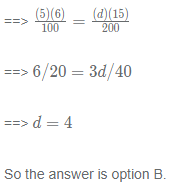RRB Group D Mock Test - 33 - Question 63

Find the speed of the flight if it takes 6 hrs 30 mins to travel 2,210 km ?

Detailed Solution for RRB Group D Mock Test - 33 - Question 63

6 hrs 30 mins = 6.5 hrs

Speed = \frac{distance}{time} = \frac{2210}{6.5} = 340 kmphtimedistance​=6.52210​=340kmph

SO the answer is option D.

RRB Group D Mock Test - 33 - Question 64

Two trains X and Y are travelling in the same direction. Their lengths are 850m and 950m respectively. They took 6 mins to cross each other. Find the speed of train X if the speed of train Y is 90 kmph ?

Detailed Solution for RRB Group D Mock Test - 33 - Question 64

Let the speed of train X is x kmph

Relative speed = 90-x kmph (if train Y is faster than train X)

Speed = distance/time = (850+950)/(6*60) = 1800/360 = 5 m/s = 5*(18/5) = 18 kmph

90 - x = 18 kmph

x = 90 - 18 = 72 kmph

So the answer is option A.

Case 2 :(If train Y is slower than train X)

x - 90 = 18, then x = 90 + 18 = 108 kmph

RRB Group D Mock Test - 33 - Question 65

A shopkeeper mixed two type of tea powders, type 1 and type 2, in the ratio 1:2 and sold the mixture at 20/- per kg. Find the profit percentage, if the cost price of type 1 and type 2 powders are 12/- and 18/- per kg respectively ?

Detailed Solution for RRB Group D Mock Test - 33 - Question 65

Let the quantity of Type 1 = k and that of Type 2 = 2k

Let x be the cost price of the mixture,

k(12)+2k(18) = (2k+k)(x)

12k+36k = 3kx

48k = 3kx

16 = x

CP = 16/- and SP = 20/-

Profit = SP - CP = 20 - 16 = 4/-

Profit % = \frac{4}{16}\times 100 = 25164​×100=25%

So the answer is option C.

RRB Group D Mock Test - 33 - Question 66

Find the LCM and HCF of 196 and 392 ?

Detailed Solution for RRB Group D Mock Test - 33 - Question 66

Here 392 = 2*196, Hence LCM is 392 and HCF is 196

So the answer is option B.

RRB Group D Mock Test - 33 - Question 67

In a moderately skewed distribution, it is known that the median is 5 and the mode is 7. What is the mean?

Detailed Solution for RRB Group D Mock Test - 33 - Question 67

Mode = 3(Median)-2(Mean)

7 = 3(5)-2(mode)

7-15 = -2(mode)

-8 = -2(mode)

mode = 4

So the answer is option D.

RRB Group D Mock Test - 33 - Question 68

576.78+456.87-x = 658.65, Find x ?

Detailed Solution for RRB Group D Mock Test - 33 - Question 68

576.78+456.87-x = 658.65

1033.65 - x = 658.65

x = 1033.65 - 658.65

x = 375

So the answer is option A.

RRB Group D Mock Test - 33 - Question 69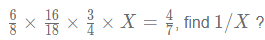Detailed Solution for RRB Group D Mock Test - 33 - Question 69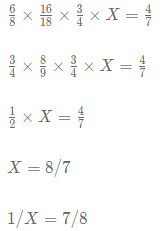So the answer is option B.

RRB Group D Mock Test - 33 - Question 70

15÷3+2×4−2=x+1, find xx ?

Detailed Solution for RRB Group D Mock Test - 33 - Question 70

15\div 3 + 2 \times 4 - 2 = x + 115÷3+2×4−2=x+1

5 +8 - 2 = x + 15+8−2=x+1

11 = x + 111=x+1

x = 10x=10

So the answer is option B.

RRB Group D Mock Test - 33 - Question 71

Find the profit percentage, if a shopkeeper earns profit equal to the selling price of 3 pens on selling 10 pens ?

Detailed Solution for RRB Group D Mock Test - 33 - Question 71

Let SP of 1 pen = 10/-

SP of 10 pens = 100/-

Profit = SP of 3 pens = 30/-

CP = SP - Profit = 100 - 30 = 70/-

Profit % = \frac{30}{70}\times 100 = 42.857030​×100=42.85%

So the answer is option A.

RRB Group D Mock Test - 33 - Question 72

Find the value of xx (from the given options), if 5x2−13x−6=0 ?

Detailed Solution for RRB Group D Mock Test - 33 - Question 72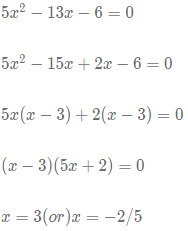So the answer is option D.

RRB Group D Mock Test - 33 - Question 73

Find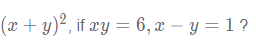Detailed Solution for RRB Group D Mock Test - 33 - Question 73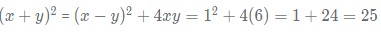So the answer is option B.

RRB Group D Mock Test - 33 - Question 74

Find the number of diagonals of a polygon of 14 sides ?

Detailed Solution for RRB Group D Mock Test - 33 - Question 74

Number of diagonals =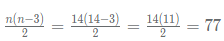So the answer is option A.

RRB Group D Mock Test - 33 - Question 75

Find the point at which x=y and x+y =0 lines meet ?

Detailed Solution for RRB Group D Mock Test - 33 - Question 75

x = y ==> x-y = 0 -----(1)

x+y = 0 ----(2)

on solving (1) and (2) we will get x = y = 0

So the answer is option A.

RRB Group D Mock Test - 33 - Question 76

The ratio of the present ages of A and B is 1:2. The sum of their ages 10 years from now will be 53. How old was A 10 years earlier?

Detailed Solution for RRB Group D Mock Test - 33 - Question 76

Let present age of A = x then that of B = 2x

Sum of their ages after 10 years = 53

(x+10)+(2x+10) = 53

3x = 33

x = 11

Present age of A = x = 11

Age of A before 10 years = x-10 = 11-10 = 1

So the answer is option D.

RRB Group D Mock Test - 33 - Question 77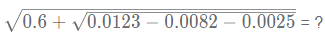Detailed Solution for RRB Group D Mock Test - 33 - Question 77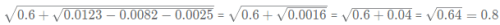So the answer is option C.

RRB Group D Mock Test - 33 - Question 78

A watch gains 6 minutes in one hour and was set right at 8 AM. What time will it show at 6PM on the same day?

Detailed Solution for RRB Group D Mock Test - 33 - Question 78

8AM to 6PM, 10 hrs

watch gains 6 minutes in one hour,

In 10hrs it gains 10*6 = 60 minutes

Hence at 6PM, it shows 6PM+60mins = 7PM

So the answer is option B.

RRB Group D Mock Test - 33 - Question 79

Find 80% of 40% of 150% of 2000 ?

Detailed Solution for RRB Group D Mock Test - 33 - Question 79

80% of 40% of 150% of 2000 = (0.8)(0.4)(1.5)(2000) = (0.32)(3000) = 960

So the answer is option C.

RRB Group D Mock Test - 33 - Question 80

Find the sum of compound interest and simple interest for 2 years on 2000/- at 10% per annum ?

Detailed Solution for RRB Group D Mock Test - 33 - Question 80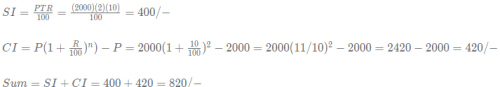So the answer is option A.

RRB Group D Mock Test - 33 - Question 81

Two taps A and B can fill a bucket in 12 hours and 15 hours respectively. If both are opened and A is closed after 3 hours, how much more time would it take for B to fill the bucket?

Detailed Solution for RRB Group D Mock Test - 33 - Question 81

Let the capacity of tank = LCM of 12 and 15 = 60 units

Efficiency of tap A = 60/12 = 5 units/hr

Efficiency of tap B = 60/15 = 4 units/hr

If both the taps are opened for 3 hrs, part of the tank that would be filled = (5+4)*3 = 27 units

Remaining part = 60 - 27 = 33 units

Tap B alone can fill 33 units of tank in 33/4 = 8\frac{1}{4} hrs = 8 hrs 15 mins841​hrs=8hrs15mins

So the answer is option D.

RRB Group D Mock Test - 33 - Question 82

Choose the incorrect number in the given series:6, 13, 27, 55, 111, 221

Detailed Solution for RRB Group D Mock Test - 33 - Question 82

The logic used is as follows:

6*2 + 1 = 12+1 = 13
13*2 + 1 = 26+1 = 27
27*2 + 1 = 54 + 1 = 55
55*2 + 1 = 110+1 = 111
111*2 + 1 = 222+1 =223

As we can see, 221 is the incorrect term in the series. Therefore, option D is the right answer.

RRB Group D Mock Test - 33 - Question 83

Choose the incorrect number in the given series:64, 729, 4096, 16625, 46656

RRB Group D Mock Test - 33 - Question 84

Choose the incorrect number in the series:31, 37, 41, 43, 47, 51, 53

Detailed Solution for RRB Group D Mock Test - 33 - Question 84

The series lists all the prime numbers from 31 in ascending order.
51 is not a prime number. It is divisible by 17. Therefore, 51 is the incorrect term in the series.

RRB Group D Mock Test - 33 - Question 85

In a certain language ‘word letter write’ is coded as ‘gw qk nc’’box write word’ is coded as ‘nc mr qk’What is the code for ‘box’?

Detailed Solution for RRB Group D Mock Test - 33 - Question 85

‘word letter write’ is coded as ‘gw qk nc’
’box write word’ is coded as ‘nc mr qk’
From the statements, we can say that the code for word/write is nc/qk and thus, code for box will be mr.
Hence, option D is the correct choice.

RRB Group D Mock Test - 33 - Question 86

In a certain language
‘call know before’ is coded as ‘gv qe nw’
’look before know’ is coded as ‘qe ml gv’
What is the code for ‘call’?

Detailed Solution for RRB Group D Mock Test - 33 - Question 86

call know before’ is coded as ‘gv qe nw’
’look before know’ is coded as ‘qe ml gv’
From the statements, we can say that the code for know/before is qe/gv. So the code for the ‘call’must be ‘nw’.
Hence, option C is the correct choice.

RRB Group D Mock Test - 33 - Question 87

In a language,
’Cars are blue roses’ is coded as ‘ta de ru la’
’ Roses are red’ is coded as ‘la ru fa’.
’red are blue’ is coded as ‘fa ru de’,

What is the code for ‘cars’?

Detailed Solution for RRB Group D Mock Test - 33 - Question 87

‘are’ is common to all the 3 sentences. Therefore, the code for ‘are’ must be ‘ru’.
’roses’ and ‘are’ common to the first 2 sentences. Therefore, the code for ‘roses’ must be ‘lu’.
’are’ and ‘blue’ are common to the first and the third sentence. Therefore, the code for ‘blue’ must be ‘de’.
We know the codes for ‘are’, ‘blue’ and ‘roses’. Therefore, from the first sentence, the code for cars must be ‘ta’. Therefore, option A is the right answer.

RRB Group D Mock Test - 33 - Question 88

Choose the option which should replace ‘?’.
B, C, E, G, K, M, ?

Detailed Solution for RRB Group D Mock Test - 33 - Question 88

The alphabets corresponding to consecutive primes are written.

B = 2
C = 3
E = 5
G = 7
K = 11
M = 13
Q = 17

RRB Group D Mock Test - 33 - Question 89

Find the word which should replace (?), so that the second pair shares the same relation as the first pair.Wealth : Coffer :: ? : Coffin

Detailed Solution for RRB Group D Mock Test - 33 - Question 89

Wealth is kept in a coffer. Similar relationship is shared by cadaver (corpse) and coffin. Hence, option B is the correct answer.

RRB Group D Mock Test - 33 - Question 90

Find the word which should replace (?), so that the second pair shares the same relation as the first pair.Ephemeral : Perineal :: Chivalrous : ?

Detailed Solution for RRB Group D Mock Test - 33 - Question 90

Ephemeral means lasting for a short time. Perineal means lasting forever. The words are antonyms of each other.
Similarly,
Chivalrous means well-mannered. Hence, rude is the antonym. Option A is the correct answer.

RRB Group D Mock Test - 33 - Question 91

Find the option which should replace (?), so that the second pair shares the same relation as the first pair.EL : I :: ? : B

Detailed Solution for RRB Group D Mock Test - 33 - Question 91

The sum of the first pair is subtracted from 26 and the corresponding letter is written.
EL : (26 - (E + L))
EL : (26 - (17))
EL : 9

Similarly,
DT : (26 - (4 + 20)
DT : B

RRB Group D Mock Test - 33 - Question 92

A statement is given below. Check which of the following conclusions logically follows the statement given and choose the appropriate option:

The investigators of the scam recently unearthed the fact that even persons with impaired visions have been issued the license to drive by the corrupt officials.

Conclusion 1: Issuing licenses for people with impaired vision is a recent phenomenon.
Conclusion 2: People with impaired vision are not supposed to be issued a driving license.

Detailed Solution for RRB Group D Mock Test - 33 - Question 92

From the statement, we can infer that people with impaired vision are not supposed to be issued driving licenses. Therefore. Conclusion 2 definitely follows.

Conclusion 1 states that the phenomenon is recent. However, the statement only states that the unearthing of the scam is recent. We do not know how long the scam has been going on. Therefore, conclusion 1 cannot be said to follow the given statement. Therefore, option B is the right answer.

RRB Group D Mock Test - 33 - Question 93

A statement is given below. Check which of the following conclusions logically follows the statement given and choose the appropriate option:

Activists are protesting against the construction of dam since the lack of silt in the region after the dam will greatly reduce the yield of the region.

Conclusion 1: Silt is an essential element for crops to grow well.
Conclusion 2: Construction of dam obstructs the flow of silt in the river.

Detailed Solution for RRB Group D Mock Test - 33 - Question 93

In the statement, it has been given that the lack of silt will reduce the yield. Therefore, we can infer that silt is an essential element for crops. Conclusion 1 follows.

The statement mentions that there will be lack of silt only in the regions ’after’ the dam. Therefore, we can infer that the dam obstructs the flow of silt. Conclusion 2 follows.

Both the conclusions follow. Therefore, option C is the right answer.

RRB Group D Mock Test - 33 - Question 94

A statement is given below. Check which of the following conclusions logically follows the statement given and choose the appropriate option:

To protect the interests of the farmers, the government has proposed to construct cold storage facilities across the country. This will ensure that farmers do not give in to market pressures and can sell their produce at a nominal price, as they will not have to worry anymore about their goods getting perished.

Conclusion 1: Earlier, farmers gave in to market pressure since their goods were perishable.
Conclusion 2: Cold storage will ensure that farmers exploit the market and not the other way around.

Detailed Solution for RRB Group D Mock Test - 33 - Question 94

From the given statement, we can infer that farmers are being exploited since their produce is perishable. The line ‘as they will not have to worry anymore about their goods getting perished.
’ implies that the goods getting perished is what that forced the farmers to accept sub par prices. Therefore, conclusion 1 follows.

Conclusion 2 states that farmers will exploit the market from now on. ‘Exploit’ is a strong word to use in this context. The given paragraph only states that farmers can fix a nominal price now which by no means can be equated to exploiting the market. Therefore, conclusion 2 does not follow.

Only conclusion 1 follows. Therefore, option A is the right answer.

RRB Group D Mock Test - 33 - Question 95

Find the odd one out.

Detailed Solution for RRB Group D Mock Test - 33 - Question 95

Except for constable, all others are related to a school. Hence, option B is the correct answer.

RRB Group D Mock Test - 33 - Question 96

Find the odd one out.

Detailed Solution for RRB Group D Mock Test - 33 - Question 96

Except for cricket, all others are Olympic games. Hence, option C is the correct answer.

RRB Group D Mock Test - 33 - Question 97

If 47 & 71 = 118 and 173 ! 48 = 125, then what is the value of 84 ! 37 & 55?

Detailed Solution for RRB Group D Mock Test - 33 - Question 97

84 ! 37 & 55 = 84 - 37 + 55 = 102
Hence, option D is the right answer.

RRB Group D Mock Test - 33 - Question 98

Arun started walking from his home. After walking 50 m, he takes a left turn and walks 60 m. Then, he takes a right turn and walks 50 m. Then, he takes a right turn again, walks 20 m, and takes a right turn. He walks 5 m. Finally, he takes a left turn and walks 15 m to enter a park through its eastern entrance. What direction was Arun facing initially when he started from his house?

Detailed Solution for RRB Group D Mock Test - 33 - Question 98

The question asks only for the direction. Therefore, the distance travelled is immaterial.
The given conditions can be expressed diagrammatically as follows: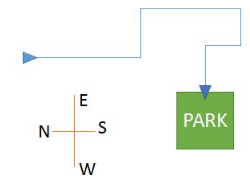As we can see, Arun must have faced the South initially. Therefore, option B is the right answer.

RRB Group D Mock Test - 33 - Question 99

Pointing to a photograph, Ranjani said that the person in the photo is the father of the son of her sister’s mother’s brother. How is the person in the photograph related to Ranjani?

Detailed Solution for RRB Group D Mock Test - 33 - Question 99

Ranjani’s bother’s mother’s sister is Ranjani’s aunt. The person in the photograph is the father of the son of this person. Therefore, the person in the photo must be the husband of Ranjani’s aunt. Therefore, the person in the photograph is the uncle of Ranjani. Hence, option D is the right answer.

RRB Group D Mock Test - 33 - Question 100

Which of the following Venn diagram captures the relationship between feminists, fathers, singers, and daughters ?

Detailed Solution for RRB Group D Mock Test - 33 - Question 100

Fathers and daughters must be represented by 2 non-intersecting circles. Singers and feminists must intersect each other, as well as fathers and daughters. Singers and feminists must also contain some space outside daughters and fathers to indicate people who are neither fathers nor daughters but are feminists or singers.

The figure must contain 2 non-intersecting circles and 2 other circles that intersect all the other 3 circles. Only option B satisfies this condition and hence, option B is the right answer.

## RRB (Group D) - Mock Tests & Previous Year Papers

1 docs|48 tests
 Use Code STAYHOME200 and get INR 200 additional OFF Use Coupon Code
Information about RRB Group D Mock Test - 33 Page
In this test you can find the Exam questions for RRB Group D Mock Test - 33 solved & explained in the simplest way possible. Besides giving Questions and answers for RRB Group D Mock Test - 33, EduRev gives you an ample number of Online tests for practice

1 docs|48 tests